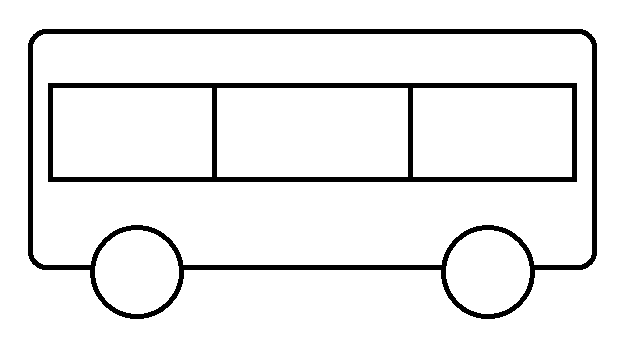## Iron 59

My father is a chemist.
My mother is a mathematician.
They call me
“Iron59”
What is my real name?

Similar Question

A mathematician and a chemist had a baby. They named their baby IRON59. What would the baby’s name be if translated into terms used in the parents’ professions?

[collapse]

Felix, because IRON in chemistry is FE and 59 in Roman numerals is LIX.

[collapse]

## Intelligence Test #7

If:

③⑤⑥ = 151872
⑤⑤⑥ = 253094
⑤⑥⑦ = 303585
⑤⑤③ = 251573

Then:

⑨④⑦ = ??????

366329

[collapse]
Explanation

ⒶⒷⒸ = [A.B][A.C][reverse(A.B+A.C-C)]

So:

⑨④⑦ = [9.4][9.7][reverse(9.4+9.7-7)]
⑨④⑦ = [reverse(92)]
⑨④⑦ = 
⑨④⑦ = 366329

[collapse]

## What Am I to Teresa

If Teresa’s daughter is my daughter’s mother, what am I to Teresa?

a. Grandmother
b. Mother
c. Daughter
d. Granddaughter
e. I am Teresa

c. Daughter

[collapse]
Explanation

Teresa’s daughter is my daughter’s mother.

My daughter’s mother is actually me (i.e. the person who is asking the puzzle).

Therefore:

Teresa’s daughter is me (i.e. the person who is asking the puzzle is Teresa’s daughter).

Note: As there are only females available for the answer, the answer cannot be a male.

[collapse]

## How Warm Will It Be?

If the temperature today is zero degrees and tomorrow the temperature is going to be twice as warm, how warm will it be?

The Fahrenheit and Celsius temperature scales are “relative” temperature scales, in that they are both relative to a couple of specific temperatures, namely the freezing and boiling points of water.

Let’s make the question a little more specific: “What is the temperature if it’s twice as warm as 0 degrees Celsius (the freezing point of water)?”

To answer this question we need to convert this Celsius temperature to an “absolute” temperature scale, a scale with no negative temperatures. We’ll use the Kelvin scale. 0 degrees Kelvin is the point at which all motion in matter stops; it is known as “absolute zero”. No temperature can exist that is lower than 0 degrees Kelvin. The formula to convert from Celsius to Kelvin temperature is:

K = C + 273.15

Where K is the Kelvin temperature and C is the Celsius temperature.

So 0 degrees Celsius is equal to 273.15 degrees Kelvin. We can double this number to get 546.30, the temperature that is twice as warm as 0 degrees Celsius. We can then subtract 273.15 from our answer to convert it back to the Celsius scale.

The temperature is 273.15 degrees Celsius when it is twice as warm as 0 degrees Celsius.

[collapse]

## Which Way the Bus Is Going?

Right or Left?Can’t you find?

Look at the picture again.

Still don’t know?

This question was asked to children of preschool in the USA with the same picture.

90% of them responded that the bus was going to left.

When asked, “Why?” they said, “Because you can’t see the door to get on the bus.”

How do you feel now?

I know, me too.

[collapse]

## Masalah 3 Buah Gelas

Diberikan 3 buah gelas. Gelas pertama memiliki kapasitas 5 liter dan berisi air 3 liter. Gelas kedua memiliki kapasitas 7 liter dan berisi air 4 liter. Gelas ketiga memiliki kapasitas 9 liter dan berisi air 5 liter.

Definisikan satu langkah penuangan sebagai berikut, “Memilih gelas A dan menuang isinya ke gelas B hingga salah satu kondisi ini terpenuhi: 1) Air di gelas A habis. 2) Air di gelas B penuh.”

Berapa banyak langkah penuangan minimal yang diperlukan untuk mendapatkan dua buah gelas yang masing-masing berisi air 6 liter?

Solusi

5 langkah.

Penjelasan:

 # Aksi Gelas A (5L) Gelas B (7L) Gelas C (9L) 0 – 3L 4L 5L 1 Tuang Gelas A ke C 0L 4L 8L 2 Tuang Gelas B ke A 4L 0L 8L 3 Tuang gelas C ke B 4L 7L 1L 4 Tuang Gelas B ke A 5L 6L 1L 5 Tuang Gelas A ke C 0L 6L 6L

[collapse]

Disumbang oleh J.P. (ITB 2008)

## If Yesterday Was Tomorrow

Samuel said, “If yesterday was tomorrow, today would be Friday.”

What day did Samuel say this?

Sunday or Wednesday depends on how you interpret this sentence, “If yesterday was tomorrow.”

[collapse]
Explanation

Let:

YES = Real Yesterday
YES’ = Imaginary Yesterday

TOD = Real Today
TOD’ = Imaginary Today

TOM = Real Tomorrow
TOM’ = Imaginary Tomorrow

Assumption I:

1. Yesterday in the sentence is the Real Yesterday (YES).
2. Tomorrow in the sentence is the Imaginary Tomorrow (TOM’).
3. Today in the sentence is the Imaginary Today (TOD’).

Thus:

YES = TOM’ (i)

TOD’ = Friday (ii)

TOM’ = TOD’ + 1 (iii)

TOD = YES + 1 (iv)

Using equation (i) and (iii):
YES = TOM’
YES = (TOD’ + 1)
YES = TOD’ + 1 (v)

Using equation (iv) and (v):
TOD = YES + 1
TOD = (TOD’ + 1) + 1
TOD = TOD’ + 2 (vi)

Using equation (ii) and (vi):
TOD = TOD’ + 2
TOD = Friday + 2
TOD = Sunday

Assumption II:

1. Yesterday in the sentence is the Imaginary Yesterday (YES’).
2. Tomorrow in the sentence is the Real Tomorrow (TOM).
3. Today in the sentence is the Imaginary Today (TOD’).

Thus:

YES’ = TOM
ATOM = YES’ (i)

TOD’ = Friday (ii)

YES’ = TOD’ – 1 (iii)

TOD = TOM – 1 (iv)

Using equation (i) and (iii):
TOM = YES’
TOM = (TOD’ – 1)
TOM = TOD’ – 1 (v)

Using equation (iv) and (v):
TOD = TOM – 1
TOD = (TOD’ – 1) – 1
TOD = TOD’ – 2 (vi)

Using equation (ii) and (vi):
TOD = TOD’ – 2
TOD = Friday – 2
TOD = Wednesday

Q.E.D.

[collapse]